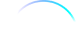## FANDOM

86,308 Pages

Though mathematical formulae are rarely needed on a wiki like this, the following help file should help you understand how to reproduce them.

Math formulas can be displayed on articles using the $ tag. For the technically inclined, MediaWiki (which is used by Fandom wikis) uses a subset of AMS-LaTeX markup, a superset of LaTeX markup which is in turn a superset of TeX markup, for mathematical formulas. ## Step by step • Open source editing mode for an article. • Write your math code in the following format: [itex]math to be displayed$.
• For example, the equation '3 x 2 = 6' can be displayed using:
${3 ~ \times ~ 2 ~ = ~ 6}$
• Which generates this:
${3 ~ \times ~ 2 ~ = ~ 6}$
• For details on how to write the math formulas themselves, see Help:Displaying a formula, on Wikipedia.
Community content is available under CC-BY-SA unless otherwise noted.